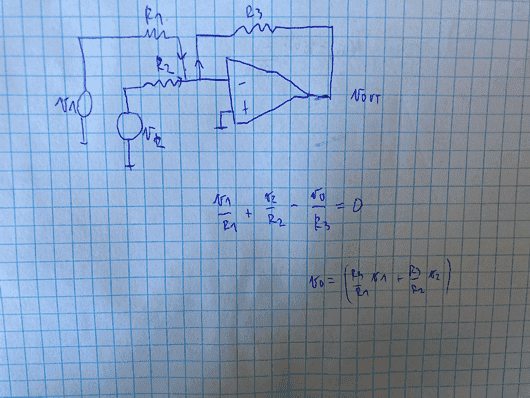# Opamp adder circuit: Where does the "-" come from?

• Engineering
altruan23
Homework Statement:
I was analyzing the adder circuit and I dont understand from where does the - come in the Vout equation.
Relevant Equations:
KCL
So i used KCL and both currents are flowing into the node, and then leaving together to go to the resistor R3.
So my eq can be seen in the picture. I was looking in a book and they had a minus infront of the parantheses.
Is the current flowing from R3 into the node??Mentor
Homework Statement:: I was analyzing the adder circuit and I don't understand from where does the - come in the Vout equation.
Relevant Equations:: KCL

So i used KCL and both currents are flowing into the node, and then leaving together to go to the resistor R3.
So my eq can be seen in the picture. I was looking in a book and they had a minus infront of the parantheses.
Is the current flowing from R3 into the node??
View attachment 298767
I'm not able to read your image (in the future, please type your work into the forum using LaTeX -- see the LaTeX Guide link below the Edit window).

But intuitively, when the input voltage goes positive, the output of the opamp needs to go negative to keep it's "-" input at ground (using the "virtual ground" property of an opamp with its "+" input at ground). Does that make any sense to you?

•altruan23
Gold Member
When you make an equation from KCL at a node, you must be careful to be consistent with the polarities of the currents. Try again with the following rule: All currents flowing into a circuit node sum to zero.

•altruan23 and berkeman
altruan23
isnt it that the sum of the currents flowing into the node, is the same as the sum of the currents flowing out of the node. and schouldnt the sum of both currents flow to the R3?

altruan23
so that means i1+i2= i3 and that is what my KCL is saying.
i set i3 as leaving the node, because there's a virtual ground so it needs to go to R3.

Gold Member
isnt it that the sum of the currents flowing into the node, is the same as the sum of the currents flowing out of the node. and schouldnt the sum of both currents flow to the R3?
Yes, you can use "into" or "out of". But your equation has some polarities as into and others out of. You must choose one version and remain consistent.

So, that "- node" is at zero volts, which we could name ## V_{neg} \equiv 0 ## (the ideal op-amp with negative feedback assumption). So, the current flowing into the that node from ##V_1 ##, ## I_1 \equiv \frac{(V_1 - V_{neg})}{R1} = \frac{(V_1)}{R1} ##. Same for all of the other currents into the that node ##I_n \equiv \frac{(V_n - V_{neg})}{Rn} = \frac{(V_n)}{Rn} ##.

If you choose the "into" rule than any current polarity that is flowing "out of" the node (as you've defined by your arrows) has to have a - sign added to correct the polarity.

Yes, the sum of ## I_1 + I_2 = I_3 ## given the polarities you've defined. This is equivalent to ## I_1 + I_2 - I_3 = 0 ## which is KCL at the ##V_{neg}## node. ## I_1 ## and ## I_2 ## flow into the node, ## I_3 ## flows out of the node, so it gets a - sign in KCL. Also, ## I_3 \equiv \frac{(V_{neg} - V_o )}{R3} = \frac{(-V_o)}{R3} ##, again with your polarity choice.

•altruan23
Mentor
so that means i1+i2= i3
I still prefer to write it as I1 + I2 + I3 = 0 with all of the current arrows pointing into the node. That helps me to avoid making brain-fade type of mistakes.

•altruan23 and DaveE
••